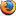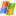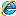### Perl 的四捨五入

```sub round{
my(\$value, \$rank) = @_;
if(\$value > 0){
return (int(\$value * 10**\$rank) + 0.5) / 10**\$rank;
}
else{
return (int(\$value * 10**\$rank) - 0.4) / 10**\$rank;
}
}
print round(12.345, 2);```

round() 函式的第一個參數為欲轉換的數值，第二個參數為欲取得的小數點位數

### JavaScript 的四捨五入

```<script type='text/javascript'>
//方法一
function round1(value, rank){
if(value >= 0){
return parseInt(value * Math.pow(10, rank) + 0.5) / Math.pow(10, rank);
}
else{
return parseInt(value * Math.pow(10, rank) - 0.4) / Math.pow(10, rank);
}
}
//方法二: 使用內建的 round() 數學函數
function round2(value, rank){
return Math.round(value * Math.pow(10, rank)) / Math.pow(10, rank);
}
//方法三: 使用內建的 toFixed() 函數 (傳回字串)
function round3(value, rank){
return Number.parseFloat(value).toFixed(rank);
}
console.log(round1(12.345, 2)); // 12.35
console.log(round2(12.345, 2)); // 12.35
console.log(round3(12.345, 2)); // "12.35"
</script>```

1.• 晴樹
• 08/21. 2008 9:29上午
UsingMozilla Firefox 3.0 onWindows Vista

很不錯的文章

像有些語言提供現成的round()，其實是『四捨六入五成雙』
判斷方式就有點小差異

2.• cvictor
• 08/26. 2008 12:38上午
UsingMozilla Firefox 2.0.0.16 onWindows XP

負數的處理有點問題喔。還是要減0.5才對。

3.• 小迪
• 10/12. 2011 4:24下午
UsingInternet Explorer 8.0 onWindows XP

呵呵，0.5中间值